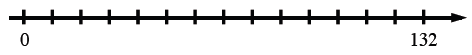### Home > CC2MN > Chapter 2 > Lesson 2.3.2 > Problem2-126

2-126.

If a set of $xy\text{‑axes}$ has $20$ grid units in each direction and the greatest value of the data to be placed on the horizontal axis is $132$, what would be an appropriate and convenient scale to use for this axis? Explain.

It may help to draw the situation. See below.Would making each interval $\frac{132}{20}$ be appropriate?

Since $\frac{132}{20}=6.6$, the best interval would be $7$